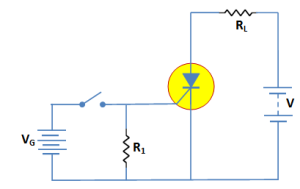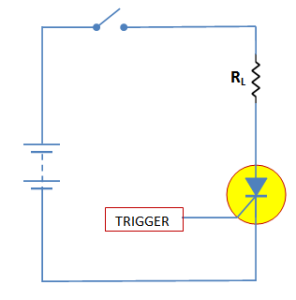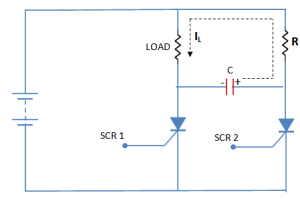# SCR as a switch

## SCR as a switch

In this article we will explain the action of an SCR as a switch in detail.

The SCR has only two states, namely; ON state and OFF state and no state inbetween.

When appropriate gate current is passed, the SCR starts conducting heavily and remains in this position indefinitelyeven if the gate voltage is removed. This corresponds to the ON condition.

However, when the anode current is reduced to the holding current, the SCR is turned OFF.

It is clear that behaviour of SCR is similar to a mechanical switch. As SCR is an electronic device, therefore, it is more appropriate to call it an electronic switch.

### SCR Switching

We have seen that SCR behaves as a switch i.e. it has only two states; ON state and OFF state.  So we will discuss the methods employed to turn on and turn off an SCR.

#### 1.   SCR turn-on methods

In order to turn on the SCR, the gate voltage Vis increased upto a minimum value to initiate triggering.

This minimum value of gate voltage at which SCR is turned ON is called gate triggering voltage VGT.

The resulting gate current is called gate triggering current IGT.

Thus to turn on an SCR all that we have to do is to apply positive gate voltage equal toVGT or pass a gate current equal to IGT.

For most of the SCRs, VGT = 2 to 10 V and IGT = 100 μA to 1500 mA.

We will discuss two methods to turn on an SCR.

##### (i) D.C. gate trigger circuit

Fig..1 shows a typical circuit used for triggering an SCR with a d.c. gate bias.Fig.1

When the switch is closed, the gate receives sufficient positive voltage (=VGT) to turn the SCR on.

The resistance Rconnected in the circuit provides noise suppression and improves the turn-on time.

The turn-on time primarily depends upon the magnitude of the gate current.

The higher the gate-triggered current, the shorter the turn-on time.

##### (ii) A.C. trigger circuit

An SCR can also be turned on with positive cycle of a.c. gate current. Fig. 2 (ii) shows such a circuit. During the positive half-cycle of the gate current, at some point =, the device is turned on as shown in fig..2 (i).

Fig.2(i)                                                                                                                                               Fig.2(ii)

#### 2.   SCR turn-off methods

The SCR turn-off poses more problem than SCR turn-on.

It is because once the device is ON, the gate loses all control. There are many methods of SCR turn-off but only two will be discussed.

##### (i)  Anode current interruption

When the anode current is reduced below a minimum value called holding current, the SCR turns off. The simple way to turn off the SCR is to open the line switch S as shown in fig.3.Fig.3

##### (ii)  Forced commutation

The method of discharging a capacitor in parallel with an SCR to turn off the SCR is called forced commutation.

Fig.4 shows the forced commutation of SCR where capacitor C performs the commutation.Fig.4

Assuming the SCRs are switches SCR1 ON and SCR2 OFF, current flows through the load and C as shown in fig.4.

When SCR2 is triggered ON, C is effectively paralleled  across SCR1.

The charge on C is then opposite to SCR1’s forward voltage, SCR1 is thus turned OFF and the current is transferred to R-SCR2 path.

You Might Like The Following Articles